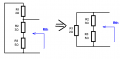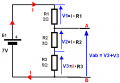#### Ryuusei_Lou

Joined Dec 14, 2014
9
I just need some guidance in comprehending how things get along in Thevenin. I'm sorry if my picture for the problem is crappy, but it's kind of bit in a rush.It is what I remembered from my professor in Circuits. I was clueless in getting the answer because the load to cut-off is at the middle. I haven't done anything yet, I just want to know the steps in getting the answer. Although, I do know how to get the answer when the load to cut-off is at the rightmost part.

#### Ryuusei_Lou

Joined Dec 14, 2014
9
Okay, I'll try. But err, question. Will there be a current flow at 3 ohms and 5 ohms when you cut-off the load?

#### Jony130

Joined Feb 17, 2009
4,974
Will there be a current flow at 3 ohms and 5 ohms when you cut-off the load?
If load is 8 ohms resistor, the answer is yes, the current will flow through 3 ohms and 5 ohms resistors after you cut-off the load.

#### Ryuusei_Lou

Joined Dec 14, 2014
9
This is where I get confused. In getting VTH, how do you look at the circuit to know what resistors to include? Sorry to ask but what could've been the general formula for VTH? I really didn't get it much when it was the lesson.

#### Ryuusei_Lou

Joined Dec 14, 2014
9
This is how I did it:

By cutting off 8 ohms, I get the VTH by this formula:

VTH = 7(5/2+3+5)
= 3.5V

Then shortening off the source, I get the RTH like this:

RTH = 2+3+5
= 8 ohms

I think it was in series so I just add them all. Then getting Vy:

Vy = 3.5(8) / 8 + 8
= 1.75V

I don't know if I'm right or not. o.o

#### Jony130

Joined Feb 17, 2009
4,974
how do you look at the circuit to know what resistors to include?
You need to include all resistors that's left after you remove the load.
Sorry to ask but what could've been the general formula for VTH?
No general formula for Vth exist.

By cutting off 8 ohms, I get the VTH by this formula:

VTH = 7(5/2+3+5)
= 3.5V
Wrong, 3.5V is a voltage across 5 ohm resistor not Vth voltage.

Then shortening off the source, I get the RTH like this:

RTH = 2+3+5
= 8 ohms
I think it was in series so I just add them all
Wrong again. Are you sure that all resistor are connect in series?#### Ryuusei_Lou

Joined Dec 14, 2014
9
Oh yay. I've got wrong answers. xD
For VTH, should it be on the left side or right side?
So for RTH, it changes position?
From what PoV should I base on? Or PoV is only needed at RTH? You know, looking at the leftmost part of the load.

#### Jony130

Joined Feb 17, 2009
4,974
Oh yay. I've got wrong answers. xD
For VTH, should it be on the left side or right side?
left/right said? Of what side?

Simply find Vy voltage for this circuit
Can you do that ?

So for RTH, it changes position?
From what PoV should I base on? Or PoV is only needed at RTH? You know, looking at the leftmost part of the load.
To find Rth all you need is to find equivalent resistance seen from "load" PoV.

#### Ryuusei_Lou

Joined Dec 14, 2014
9
I think I'm gonna need some hammer. (>.>) What I mean is, looking at the circuit; should I include 2 which was on the left of 8 (the load) or 3 and 5? o.o

Anyways, I'm still guessing about VTH:
7(8/3+5) = 1v?
7(5/3+5) = 4.38v?

For RTH:
2(8)/2+8 = 1.6 ohms

#### Jony130

Joined Feb 17, 2009
4,974
I think I'm gonna need some hammer. (>.>) What I mean is, looking at the circuit; should I include 2 which was on the left of 8 (the load) or 3 and 5? o.o

Anyways, I'm still guessing about VTH:
7(8/3+5) = 1v?
7(5/3+5) = 4.38v?
No, you don't need a hammer, you simply need back to the basic.
So let as start again. After we remove 8 ohm resistor from your circuit we left with this circuit

So we have a very simple circuit that contains 3 resistors connected in series. In a series circuit, the current through each of the components is the same because the current has only one path to take.
This current is equal to

I = V/R = 7V/(2Ω + 3Ω + 5Ω) = 0.7A

And this current will "create" a voltage drop across each resistor equal to V = I*R. Additional notice that we want to find Vth voltage.
And Vth voltage is a sum of a voltage drops across R2 and R3.For RTH:
2(8)/2+8 = 1.6 ohms
Looks good.

#### Ryuusei_Lou

Joined Dec 14, 2014
9
So it's:

VTH = 7/(2+3+5) = 0.7V
RTH = 1.6 Ω

Vy = 0.7(8)/8+1.6
=0.58V

What should I remember about getting VTH? o.o

#### Jony130

Joined Feb 17, 2009
4,974
Wrong.
VTH = 7/(2+3+5) = 0.7V
Check your units. Since when V/Ω is equal to volts ?

#### Ryuusei_Lou

Joined Dec 14, 2014
9
Oh sorry. Didn't see.
VTH = 5.6V from 3(0.7) = 2.1 ; 5(0.7) = 3.5
RTH = 1.6 Ω
Vy = 4.67V

#### Jony130

Joined Feb 17, 2009
4,974
Oh sorry. Didn't see.
VTH = 5.6V from 3(0.7) = 2.1 ; 5(0.7) = 3.5
RTH = 1.6 Ω
Finally you got that part right

$V_{TH} = E_1 * \frac{R_2 + R_3}{R_1+R_2+R_3} = 7V *\frac{8\Omega}{10\Omega} = 5.6V$

As for Vy = Vth * Rload/(Rth + Rload) = 5.6V * 8Ω/9.6Ω ≈ 4.7V

#### Ryuusei_Lou

Joined Dec 14, 2014
9
Thank you. I have a better understanding now.

#### WBahn

Joined Mar 31, 2012
24,692
It's a bit hard to say for sure, but from your first post and a couple of things that you've said after that, it appears that you are too focused on memorizing formulas that apply to circuits that match a very specific pattern instead of understanding the underlying concepts in such a way that allow you to apply those concepts to problems that don't match any pattern you have yet seen.

Even if you choose to continue down this path, you can increase your success rate by learning how to transform circuits from an unfamiliar pattern into a pattern that matches one you have seen before. In this case, the fact that the load is in the middle was throwing you off but you indicated that if the load was on the right that you would have known how to solve it. Okay, so ask yourself if it is possible to redraw the circuit so that the load is on the right while preserving all of the connections. What if you put the series connected 3Ω and 5Ω resistors where the 8Ω resistor originally was and put the 8Ω resistor where the 5Ω resistor was (the 3Ω resistor just gets replaced with a short)? Could you solve it now?

But far better is to learn the underlying concepts behind, in this case, the very notion of a Thevenin equivalent circuit. In short, the idea is that you can take a circuit and partition it into two parts, a 'load' and 'everything else' in such a way that the two subcircuits are connected at exactly two points (the 'terminals'). As long as everything in the 'everything else' part of the circuit is linear, you can then replace 'everything else' with an equivalent subcircuit that consists of nothing but a simple voltage source in series with resistance (limiting the discussion to circuits that only have sources and resistances now) and the 'load' part of the circuit will be unable to detect whether it is connected to the original 'everything else' subcircuit or the equivalent subcircuit. To find the equivalent subcircuit, we remove the load and find the needed parameters for the equivalent circuit (the V and the R). There are a number of ways of doing this, but in general we need two equations since we have two unknowns. By looking at the equivalent circuit, it should be fairly obvious that if we leave the terminals unconnected that the voltage that will appear across them will equal V while if we short the terminals together that the current flowing through the short will be I=V/R. These are the 'open-circuit voltage' and the 'short-circuit current', respectively. Since the two subcircuits are equivalent (as seen at the terminals), we can therefore apply this same approach to the original subcircuit to get these same parameters.

#### WBahn

Joined Mar 31, 2012
24,692
This is how I did it:

By cutting off 8 ohms, I get the VTH by this formula:

VTH = 7(5/2+3+5)
= 3.5V

Then shortening off the source, I get the RTH like this:

RTH = 2+3+5
= 8 ohms

I think it was in series so I just add them all. Then getting Vy:

Vy = 3.5(8) / 8 + 8
= 1.75V

I don't know if I'm right or not. o.o
You need to learn order of operations; multiplication and division come before addition and subtraction.

A/B+C = (A/B) + C and is NOT the same as A/(B+C)

#### WBahn

Joined Mar 31, 2012
24,692
So it's:

VTH = 7/(2+3+5) = 0.7V
RTH = 1.6 Ω

Vy = 0.7(8)/8+1.6
=0.58V

What should I remember about getting VTH? o.o
Always, always, ALWAYS track your units! Don't just tack on whatever units you WANT the answer to have to the final result. Most mistakes you make will screw up the units allowing you to catch them right away, but you can only catch then if the units are there to be screwed up.

VTH = 7V/(2Ω+3Ω+5Ω) = 7V/10Ω = 0.7A OOPS! Units are wrong, so answer is guaranteed to be wrong.